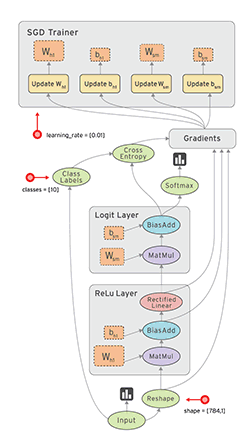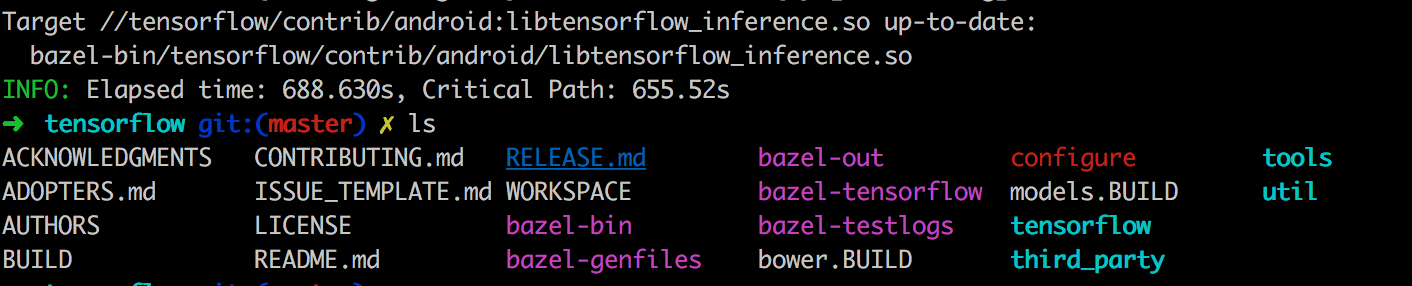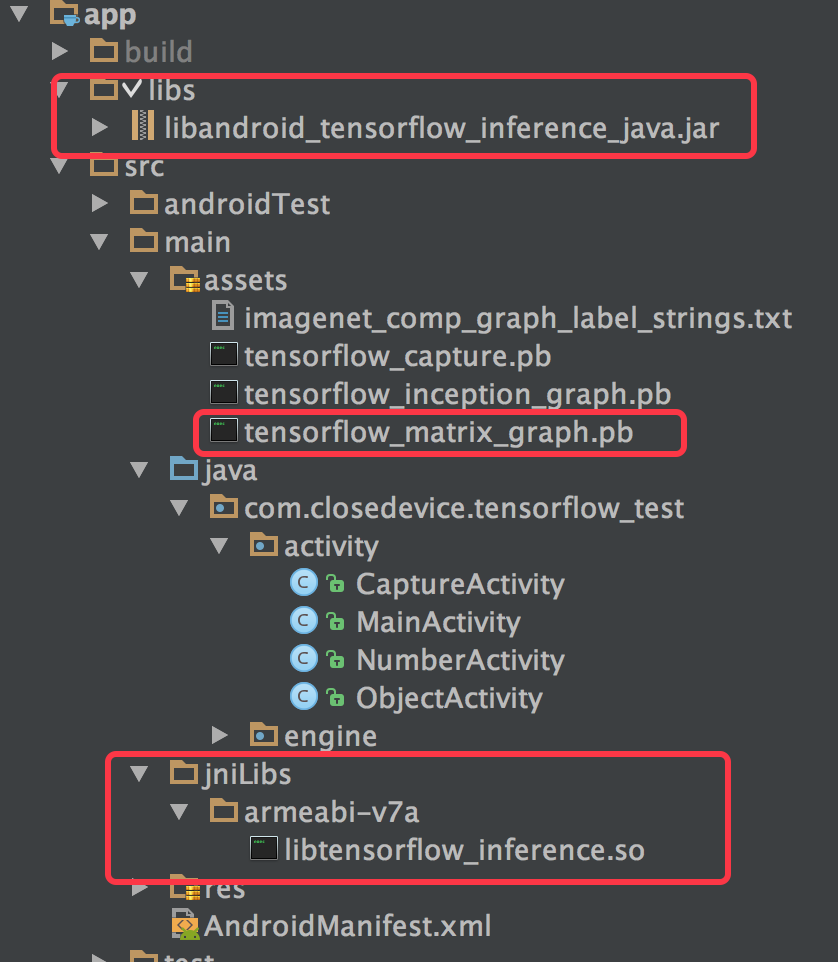# TensorFlow学习笔记(一)

## 机器学习

### 浅层学习

90年代，各种各样的浅层机器学习模型相继被提出，比如SVM、Boosting、最大熵方法等。这些模型在是理论分析或者工程应用领域都获得了巨大的成功最成功的应用,比如搜索广告系统的广告点击率CTR预估、网页搜索排序、垃圾邮件过滤系统、基于内容的推荐系统等。

### 深度学习## TensorFlow基础• 灵活性： 非严格的“神经网络”库。这意味这我们的计算只要能够表示为数据流图,就能够使用.
• 可移植性：底层核心采用C++便宜,可以运行在台式机、服务器、手机移动等设备上,提供对分布式的支持,能够快速构建深度学习集群.
• 多语言支持：前端支持Python,C/C++,Java以及Go,以及非官方支持的Scala,但是目前对Python接口支持最好.
• 高效：提供对线程、队列、异步操作支持，同时支持运行在CPU和GPU上,能够充分发挥硬件潜力.TensorFlow中的计算图就像此处的管道结构,我们考虑设计管道结构的过程就是在构建计算图.

TensorFlow使用Graph来描述计算任务,图中的节点被称之为op.一个op可以接受0或多个tensor作为输入,也可产生0或多个tensor作为输出.任何一个Graph要想运行,都必须借助上下文Session.通过Session启动Graph,并将Graph中的op分发到CPU或GPU上,借助Session提供执行这些op.op被执行后,将产生的tensor返回.借助Session提供的feed和fetch操作,我们可以为op赋值或者获取数据.计算过程中,通过变量(Variable)来维护计算状态.

Session会话图必须在称之为“会话”的上下文中执行。会话将图的op分发到诸如CPU或者GPU上计算
Graph描述计算过程必须在Session中启动
tensor数据数据类型之一,代表多维数组
op操作图中的节点被称之为op，一个op获得0或者多个Tensor，执行计算，产生0或者多个Tensor
Variable变量数据类型之一,运行过程中可以被改变,用于维护状态
feed赋值为op的tensor赋值
fetch取值从op的tensor中取值
Constant常量数据类型之一,不可变

## TensorFlow环境

TensorFlow目前支持三种平台:Linux系列,Mac OS以及Window.并提供了多种安装方式,目前常见的安装方式有三种:pip,docker,Anacona,和源码编译安装.TensorFlow支持CPU计算和GPU计算:

• CPU 支持 :系统没有 NVIDIA CUDA® GPU，我们只能安装该版本。
• GPU 支持: TensorFlow 程序通常在 GPU 比在 CPU 上运行快得多。如果系统具有 NVIDIA CUDA GPU 那么可以安装该版本

### window上通过安装

pip3 install tensorflow
pip3 install tensorflow-gpu
pip3 install tensorlayer //上面二选一,后安装tensorlayer,也可以不装

   return importlib.import_module(mname)
File "C:\Users\liudongdong-iri\AppData\Local\Programs\Python\Python35\lib\impo
ib\__init__.py", line 126, in import_module
return _bootstrap._gcd_import(name[level:], package, level)
File "<frozen importlib._bootstrap>", line 986, in _gcd_import
File "<frozen importlib._bootstrap>", line 969, in _find_and_load
File "<frozen importlib._bootstrap>", line 958, in _find_and_load_unlocked
File "<frozen importlib._bootstrap>", line 666, in _load_unlocked
File "<frozen importlib._bootstrap>", line 577, in module_from_spec
File "<frozen importlib._bootstrap_external>", line 906, in create_module
File "<frozen importlib._bootstrap>", line 222, in _call_with_frames_removed
mportError: DLL load failed: 找不到指定的模块。 

File "<frozen importlib._bootstrap>", line 222, in _call_with_frames_removed
ImportError: DLL load failed: 找不到指定的模块。

### Mac OS X 通过pi安装

pip install tensorflow

pip3 install tensorflow

### 检测安装

import tensorflow as tf

hello = tf.constant('Hello,TensorFlow')
sess = tf.Session()
print(sess.run(hello))

Hello,TensorFlow

## TensorFlow实践

import tensorflow as tf

# 定义‘符号’变量，也称为占位符
a = tf.placeholder("int32")
b = tf.placeholder("int32")

# 构造一个op节点
y = tf.multiply(a, b)

# 建立会话
sess = tf.Session()

# 运行会话，输入数据，并计算节点，同时打印结果
print(sess.run(y, feed_dict={a: 3, b: 3}))

# 任务完成, 关闭会话.
sess.close()

### 构建阶段

#### Graph

Graph:要组装的结构,由许多操作组成,其中的每个连接点代表一种操作

tf.Graph.as_graph_def()返回一个图的序列化的GraphDef,表示序列化的GraphDef可以导入到另外一个图(使用import_graph_def())
tf.Graph.get_operations()返回图中的操作节点列表
tf.Operation.name操作节点op的名称
tf.Operation.type操作节点op的类型
tf.Operation.inputs操作节点的输入与输出
tf.Operation.run(session=None,feed_dict=None)在会话中执行该操作
tf.get_collection(key,scope=None)基于默认的图,其功能便为Graph.get_collection()

#### op

op:接受（流入）零个或多个输入（液体），返回（流出）零个或多个输出

#### 数据类型

tensor:多维array或list

# 创建
tensor_name=tf.placeholder(type, shape, name)

variable:通常可以将一个统计模型中的参数表示为一组变量。例如，你可以将一个神经网络的权重当作一个tensor存储在变量中。在训练图的重复运行过程中去更新这个tensor

# 创建变量
name_variable = tf.Variable(value, name)

# 初始化单个变量
init_op=variable.initializer()

# 初始化所有变量
init_op=tf.initialize_all_variables()

# 更新操作
update_op=tf.assign(variable to be updated, new_value)

#### 简单示例

import tensorflow as tf

# 创建作为第一个常量op,该op会被加入到默认的图中
# 1*2的矩阵,构造器的返回值代表该常量op的返回值
matrix_1 = tf.constant([[3., 3.]])

# 创建第二个常量op,该op会被加入到默认的图中
# 2*1的矩阵
matrix_2 = tf.constant([[2.], [2.]])

# 创建第三个op,为矩阵乘法op,接受matrix_1和matrix_2作为输入,product代表乘法矩阵结果
product = tf.matmul(matrix_1, matrix_2)

### 执行阶段

#### Session

tf.Session.close()关闭会话
tf.Session.graph返回加载该会话的图()
tf.Session.as_default()设置该对象为默认会话,并返回一个上下文管理器

### 简单示例

# 创建会话
sess = tf.Session()

# 赋值操作
sess.run([output], feed_dict={input1:value1, input2:value1})

# 用创建的会话执行操作
sess.run(op)

# 取值操作

# 关闭会话
sess.close()

### 完整示例

import tensorflow as tf

# 创建作为第一个常量op,该op会被加入到默认的图中
# 1*2的矩阵,构造器的返回值代表该常量op的返回值
matrix_1 = tf.constant([[3., 3.]])
# 创建第二个常量op,该op会被加入到默认的图中
# 2*1的矩阵
matrix_2 = tf.constant([[2.], [2.]])
# 创建第三个op,为矩阵乘法op,接受matrix_1和matrix_2作为输入,product代表乘法矩阵结果
product = tf.matmul(matrix_1, matrix_2)
# 获取sess
sess = tf.Session()
# 来执行矩阵乘法op
result = sess.run(product)
# 输出矩阵乘法结果
print("result:",result)

# 任务完毕,关闭Session
sess.close()

with tf.Session() as sess:
result=sess.run(product)
print("result:",result)

result: [[ 12.]]

### 其他

#### Tensor

tf.Tensor.dtypetensor中数据类型
tf.Tensor.nametensor名称
tf.Tensor.op产生该tensor的op
tf.Tensor.graph该tensor所在的

#### Variables

TensorFlow使用Variables来维护图执行过程中的状态信息.下面我们演示一个计数器:

import tensorflow as tf

# 创建一个变量,初始化为0
state = tf.Variable(0, name="counter")

# 创建一个常量
one = tf.constant(1)
update = tf.assign(state, new_value)

# 变量初始化
init_op = tf.initialize_all_variables()

sess = tf.Session()
# 运行init_op
sess.run(init_op)
# 运行state,打印state初始值
print(sess.run(state))
for _ in range(10):
sess.run(update)
print(sess.run(state))

0
1
2
3
4
5
6
7
8
9
10

#### Fetch

import tensorflow as tf

value_1 = tf.constant(3.0)
value_2 = tf.constant(2.0)
value_3 = tf.constant(5.0)

# 2.0+5.0

# 3.0+(2.0+5.0)

sess = tf.Session()
print(sess.run([temp_value,result]))

#### Feed

import tensorflow as  tf

input_1 = tf.placeholder(tf.float32)
input_2 = tf.placeholder(tf.float32)

with tf.Session() as sess:
# 通过feed_dict来输入,outpu表示输出
print(sess.run([output],feed_dict={input_1:[7.],input_2:[2.]}))

#### placeholder

TensorFlow提供一种占位符操作,在执行时需要为其提供数据.这有点类似我们编写sql语句时使用?占位符一样,你可以理解为预编译.

tf.placeholder(dtype,shape=None,name=None)为一个tensor插入一个占位符
input_value = tf.placeholder(tf.float32,shape=(1024,1024))

#### 模型保存于恢复

tf.train.Saver.save(sess,save_path,global_step=None,latest_filename=None,meta_graph_suffix=’meta’,write_meta_graph=True)保存变量
tf.train.Saver.restore(sess,save_path)恢复变量
tf.train.Saver.last_checkpoints()列出最近未删除的checkpoint文件名
tf.train.Saver.set_last_checkpoints(last_checkpoints)设置checkpoint文件名列表
tf.train.Saver.set_last_checkpoints_with_time(last_checkpoints_with_time)设置checkpoint文件名列表和时间戳

import tensorflow as tf

def save_model():
v1 = tf.Variable(tf.random_normal([1, 2]), name="v1")
v2 = tf.Variable(tf.random_normal([2, 3]), name="v2")
init_op = tf.global_variables_initializer()
saver = tf.train.Saver()
with tf.Session() as sess:
sess.run(init_op)
saver_path = saver.save(sess, "./model.ckpt")
print("model saved in file: ", saver_path)

import tensorflow as tf

v1 = tf.Variable(tf.random_normal([1, 2]), name="v1")
v2 = tf.Variable(tf.random_normal([2, 3]), name="v2")
saver = tf.train.Saver()
with tf.Session() as sess:
saver.restore(sess,"./model.ckpt")
print("mode restored")


## 移植TensorFlow到移动设备

1. PC训练模型,并将其保存为pb格式,然后导入该模型文件到Android项目的assets目录中
2. 导入TensorFlow的jar包以及so文件到Android项目中,jar包向我们暴露了操作接口,具体的执行引擎算法责备封装在so文件当中
3. 定义相关变量,存储数据,并通过jar包提供的接口加载模型,执行运算即可.

### 1. 训练模型

import tensorflow as tf

sess = tf.Session()
matrix_1 = tf.constant([3., 3.], name='input')

output_graph_def = tf.graph_util.convert_variables_to_constants(sess, sess.graph_def, output_node_names=['output'])
# 保存模型到目录下的model文件夹中
with tf.gfile.FastGFile('./model/tensorflow_matrix_graph.pb',mode='wb') as f:
f.write(output_graph_def.SerializeToString())

sess.close()


1. 不能使用 tf.train.write_graph()保存模型，该种方式只是保存了模型的结构，并不保存训练完毕的参数值
2. 不能使用 tf.train.saver()保存模型，该种方式只是保存了网络中的参数值，并不保存模型的结构。

# 可以把整个sesion当作常量都保存下来，通过output_node_names参数来指定输出
graph_util.convert_variables_to_constants
# 指定保存文件的路径以及读写方式
tf.gfile.FastGFile('model/test.pb', mode='wb')
# 将固化的模型写入到文件
f.write(output_graph_def.SerializeToString())

### 2.编译所需要的jar和so文件

1. 首先克隆 TensorFlow 仓库到本地:
\$ git clone --recurse-submodules https://github.com/tensorflow/tensorflow

--recurse-submodules 参数是必须得, 用于获取 TesorFlow 依赖的 protobuf 库.

1. 安装Bazel

brew install bazel

Build label: 0.4.5-homebrew
Build time: Thu Mar 16 13:37:54 2017 (1489671474)
Build timestamp: 1489671474
Build timestamp as int: 1489671474# Uncomment and update the paths in these entries to build the Android demo.
#android_sdk_repository(
#    name = "androidsdk",
#    api_level = 23,
#    # Ensure that you have the build_tools_version below installed in the
#    # SDK manager as it updates periodically.
#    build_tools_version = "25.0.2",
#    # Replace with path to Android SDK on your system
#    path = "<PATH_TO_SDK>",
#)
#
# Android NDK r12b is recommended (higher may cause issues with Bazel)
#android_ndk_repository(
#    name="androidndk",
#    path="<PATH_TO_NDK>",
#    # This needs to be 14 or higher to compile TensorFlow.
#    # Note that the NDK version is not the API level.
#    api_level=14)

# Uncomment and update the paths in these entries to build the Android demo.
android_sdk_repository(
name = "androidsdk",
api_level = 23,
# Ensure that you have the build_tools_version below installed in the
# SDK manager as it updates periodically.
build_tools_version = "25.0.2",
# 修改为自己系统SDK路径
path = "/Users/liudongdong/Library/Android/sdk/",
)
#
# Android NDK r12b is recommended (higher may cause issues with Bazel)
android_ndk_repository(
name="androidndk",
# 修改为自己系统NDK路径
path="/Users/liudongdong/Library/Android/ndk/",
# This needs to be 14 or higher to compile TensorFlow.
# Note that the NDK version is not the API level.
api_level=14)

bazel build -c opt //tensorflow/contrib/android:libtensorflow_inference.so --crosstool_top=//external:android/crosstool --host_crosstool_top=@bazel_tools//tools/cpp:toolchain --cpu=armeabi-v7a

bazel-bin/tensorflow/contrib/android/libtensorflow_inference.so

bazel build //tensorflow/contrib/android:android_tensorflow_inference_java

bazel-bin/tensorflow/contrib/android/libandroid_tensorflow_inference_java.jar

### 3.移植模型到Android设备

1. 将pb模型文件放入assets目录

2. 添加jar包到项目的libs目录下,添加so文件到jniLibs目录下:1. 定义变量,然后初始化tensorflow,调用相关api.同样,我们以刚才生成的tensorflow_matrix_graph.pb为例:
public class NumberActivity extends AppCompatActivity {
// 定义模型文件路径
private static final String MODE_FILE = "file:///android_asset/tensorflow_matrix_graph.pb";

private static final int HEIGHT=1;
private static final int WIDTH =2;

// 输入
private static final String inputName = "input";
private float[] inputs = new float[HEIGHT * WIDTH];

// 输出
private static final String outputName = "output";
private float[] outputs = new float[HEIGHT * WIDTH];

//tensorflow接口
TensorFlowInferenceInterface mTensorFlowInferenceInterface;

@Override
protected void onCreate(Bundle savedInstanceState) {
super.onCreate(savedInstanceState);
setContentView(R.layout.activity_number);
findViewById(R.id.btn_matrix).setOnClickListener(new View.OnClickListener() {
@Override
public void onClick(View v) {
float[] result = getResult();
Toast.makeText(NumberActivity.this, result + "  " + result, Toast.LENGTH_SHORT).show();
}
});
mTensorFlowInferenceInterface = new TensorFlowInferenceInterface(getAssets(), MODE_FILE);
}

private float[] getResult() {
inputs=4;
inputs=3;

Trace.beginSection("feed");
// 输入数据
mTensorFlowInferenceInterface.feed(inputName, inputs, WIDTH, HEIGHT);
Trace.endSection();

Trace.beginSection("run");
String[] outputNames = new String[]{outputName};
// 执行数据
mTensorFlowInferenceInterface.run(outputNames);
Trace.endSection();

Trace.beginSection("fetch");
// 取出数据
mTensorFlowInferenceInterface.fetch(outputName,outputs);
Trace.endSection();

return outputs;
}
}

## 总结08-02377
12-1314万+
05-29316
01-233万+
03-221661
02-01328
01-22187
06-07334
10-14268
07-042万+
07-216359
06-024万+
03-211万+
01-09772
04-20277
09-17119
04-283311
03-13164
02-12727